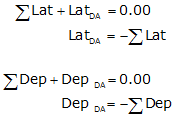## 2. Procedure

An open traverse< Figure I-2, is closed by making it meet the closure condition:Equations D-3 and D-4Figure J-2 Lats and Deps on Open Link Traverse

When the latitudes and departures are added, the amount by which they don't equal zero are the latitude and departure of the closing line, Figure I-3.Figure J-3 Lat and Dep of Closing LineEquations J-1 and J-2

Once the latitude and departure of the closing line are determined, its length and direction can be computed from equations we've used before:Equation J-3Equation J-4

The mathematical signs on the closing latitude and departure determine in which quadrant the line is.